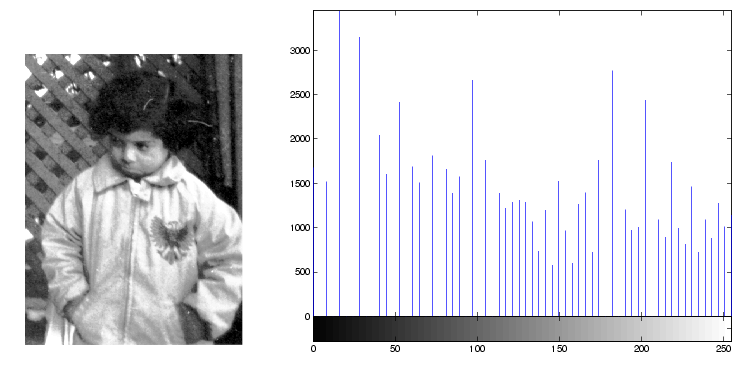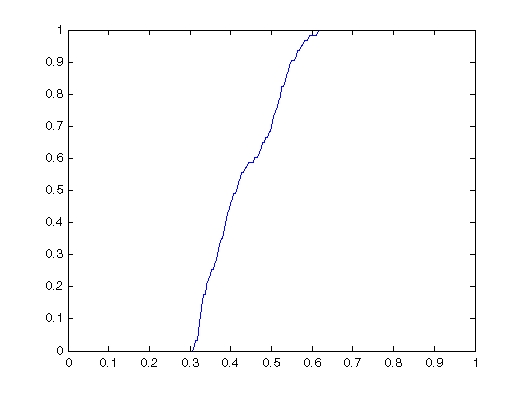Image Processing Toolbox User's GuideHistogram Equalization

The process of adjusting intensity values can be done automatically by the `histeq` function. `histeq` performs histogram equalization, which involves transforming the intensity values so that the histogram of the output image approximately matches a specified histogram. (By default, `histeq` tries to match a flat histogram with 64 bins, but you can specify a different histogram instead; see the reference page for `histeq`.)

This example illustrates using `histeq` to adjust a grayscale image. The original image has low contrast, with most values in the middle of the intensity range. `histeq` produces an output image having values evenly distributed throughout the range.

• ```I = imread('pout.tif');
J = histeq(I);
imshow(J)
figure, imhist(J,64)```

Image After Histogram Equalization with Its Histogram

`histeq` can return a 1-by-256 vector that shows, for each possible input value, the resulting output value. (The values in this vector are in the range [0,1], regardless of the class of the input image.) You can plot this data to get the transformation curve. For example:

• ```I = imread('pout.tif');
[J,T] = histeq(I);
figure,plot((0:255)/255,T);```

Notice how this curve reflects the histograms in the previous figure, with the input values mostly between 0.3 and 0.6, while the output values are distributed evenly between 0 and 1.

For an interactive demonstration of intensity adjustment, try running `imadjdemo`.Adjusting Intensity Values to a Specified Range Contrast-Limited Adaptive Histogram Equalization